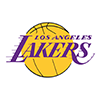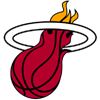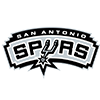PointsBet 2019 Championship Odds
+190
1.9 to 1Bucks
26.2% implied probability

+250
2.5 to 1Lakers
21.7% implied probability

+300
3 to 1Clippers
19.0% implied probability

+1200
12 to 1Rockets
5.8% implied probability

+1500
15 to 1Celtics
4.7% implied probability

+1600
16 to 1Raptors
4.5% implied probability

+2500
25 to 1Nuggets
2.9% implied probability

+2500
25 to 1Jazz
2.9% implied probability

+2500
25 to 176ers
2.9% implied probability

+3000
30 to 1Heat
2.4% implied probability

+4000
40 to 1Mavericks
1.8% implied probability

+4000
40 to 1Nets
1.8% implied probability

+8000
80 to 1Pacers
0.9% implied probability

+10000
100 to 1Thunder
0.8% implied probability

+15000
150 to 1Grizzlies
0.5% implied probability

+20000
200 to 1Pelicans
0.4% implied probability

+20000
200 to 1Trail Blazers
0.4% implied probability

+50000
500 to 1Magic
0.2% implied probability

+50000
500 to 1Spurs
0.2% implied probability

+200000
2000 to 1Wizards
0.0% implied probability